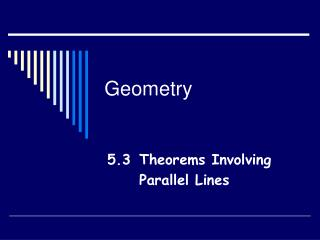DownloadDownload PresentationGeometry

# Geometry

Download Presentation## Geometry

- - - - - - - - - - - - - - - - - - - - - - - - - - - E N D - - - - - - - - - - - - - - - - - - - - - - - - - - -
##### Presentation Transcript

1. Geometry 5.3 Theorems Involving Parallel Lines

2. 4 UsefulTheorems Today you will learn 4 useful theorems about parallel lines. These will help you solve problems and write proofs.

3. Theorem If 2 lines are parallel, then all points on one line are equidistant from the other line. A B AC = BD l m C D

4. Theorem If 3 parallel lines cut off congruent segments on one transversal, then they cut off congruent segments on every transversal. If AB = BC then XY = YZ A X B Y C Z

5. Theorem A line that contains the midpoint of one side of a triangle and is parallel to another side passes through the midpoint of the third side. X If M is the midpoint of XY then N is the midpoint of XZ N M Y Z

6. Theorem A segment that joins the midpoints of 2 sides of a triangle; 1. is parallel to the third side. 2. is half as long as the third side. X • MN YZ • MN = ½YZ N M Y Z

7. A P B Q C R D S Sample Problems: • Find x when PQ = 3x – 9 and QR = 2x – 2. • Find PR and PS when PQ = 5. • Find RS when PS = 33. • Find x when PR = x + 8 and QS = 3x – 2. • 5) Find QS in problem #4.

8. X X X 6 4 3 A A B A B B 4 5 3 Y Y Z Z Y Z C C C 5 6 6 6 6) Name the points shown that MUST be midpoints of the sides of the large triangle.

9. X N M Z Y • Find YZ if MN = 8. • Find MN if YZ = 30. • Find YZ if MN = 2k + 3. • 10) Find MN if YZ = k.

10. X N M Z Y 11) Find x, MN and YZ if MN = x and YZ = 3x – 25. 12) Find if and .

11. A P B Q C R D S 13) Find x and y if AB = 19, BC = x + 3y, and CD = 6x – y.

12. Homework P. 180 #1-17

13. Let’s Try A Few Together • Practice 18 # 6 • Test 18 #2, 11, 12